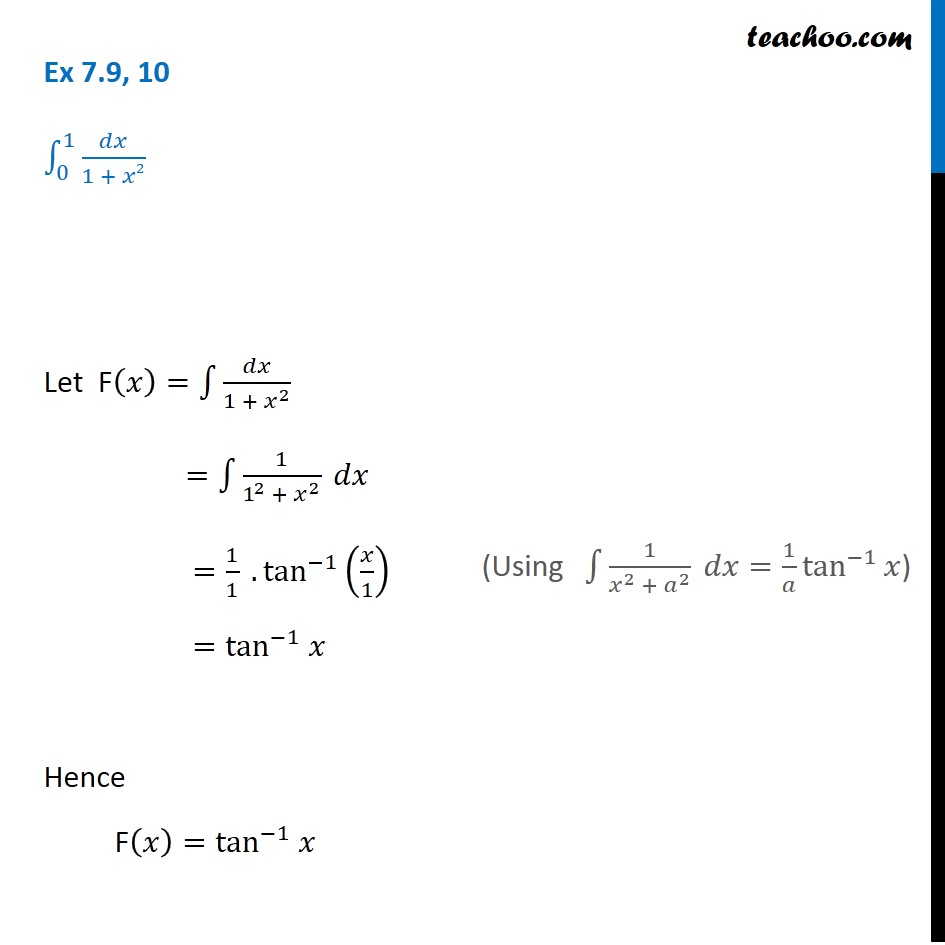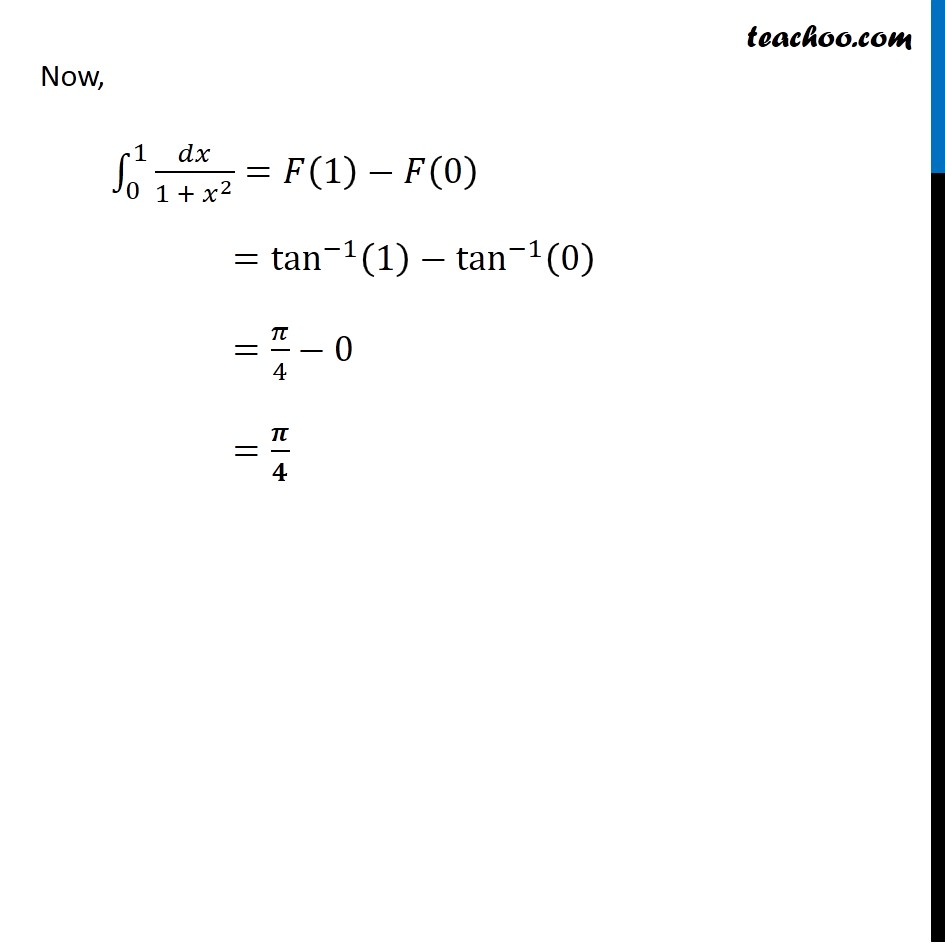Ex 7.8

Chapter 7 Class 12 Integrals
Serial order wiseLearn in your speed, with individual attention - Teachoo Maths 1-on-1 Class

### Transcript

Ex 7.8, 10 ∫_0^1▒𝑑𝑥/(1 + 𝑥2) Let F(𝑥)=∫1▒𝑑𝑥/(1 + 𝑥^2 ) =∫1▒1/(1^2 + 𝑥^2 ) 𝑑𝑥 =1/1 .tan^(−1)⁡(𝑥/1) =tan^(−1) 𝑥 Hence F(𝑥)=tan^(−1) 𝑥 (Using ∫1▒1/(𝑥^2 + 𝑎^2 ) 𝑑𝑥=1/𝑎 tan^(−1)⁡𝑥) Now, ∫_0^1▒〖𝑑𝑥/(1 + 𝑥^2 )=𝐹(1)−𝐹(0) 〗 =tan^(−1)⁡〖(1)−tan^(−1)⁡(0) 〗 =𝜋/4−0 =𝝅/𝟒